• Cartographer 2D SLAM算法,2D 的流行算法，非常不错的文档
• ros中的五种2D SLAM算法,论文对常用的五种2D slam 算法进行了全方面的比较，论文对常用的五种2D slam 算法进行了全方面的比较
• ## 激光 2DSLAM 学习

千次阅读 2018-06-11 09:41:25
激光 2D SLAM 本文主要介绍的是基于激光雷达的 2D SLAM，以及我最近看的粒子滤波、GMapping、Cartographer 等内容。 什么是 SLAM 图 1. cartographer 建的地图 全称 simultaneous localization and mapping...
激光 2D SLAM

本文主要介绍的是基于激光雷达的 2D SLAM，以及我最近看的粒子滤波、GMapping、Cartographer 等内容。

什么是 SLAM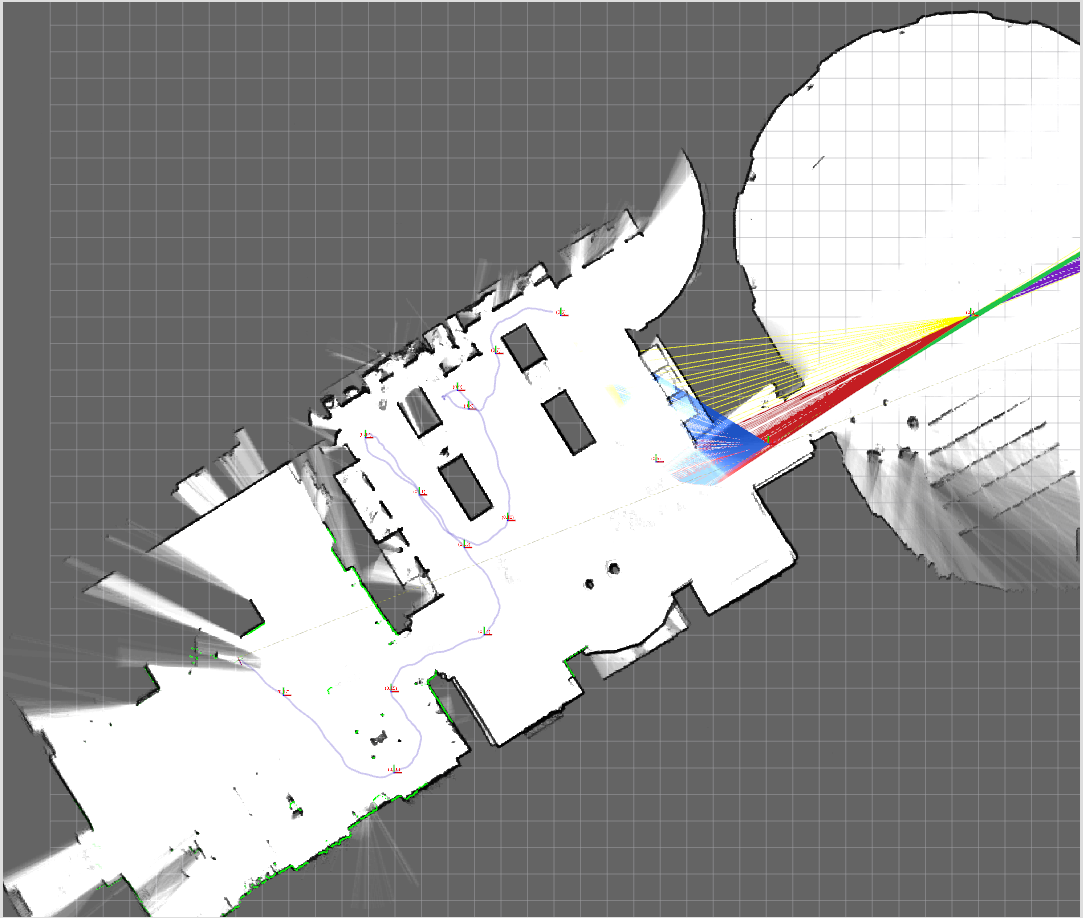图 1. cartographer 建的地图

全称 simultaneous localization and mapping，顾名思义，就是在机器人构建周围环境的地图的同时，定位它在地图中的位置。

算法的输入一般是：机器人对周围环境的观测信息 z (激光雷达的扫描点、摄像头的图像等) 和 机器人的控制信息 u (运动过程中的前进转向等信息，一般对其积分后用里程计 odometry 来表示)；

算法的输出是：机器人的运动轨迹 x 和 周围的地图 m 。

而我们要做的就是在给定 z 和 u 的条件下估计出 x 和 m：

p(x,m|z,u)$p\left(x,m|z,u\right)$p(x,m|z,u)

这里我觉得可以稍微说一下为什么要用一个条件概率以及为什么可以用一个条件概率来表示我们的问题，因为在一开始接触机器人的时候我自己就有这样的疑惑。

首先为什么要用一个条件概率而不是一个封闭式的函数？因为我们无法对整个系统精确建模，或者说精确建模的代价太高，比如说传感器自身的测量误差（一般由厂家提供）、传感器与环境交互时的误差（激光雷达遇强光或黑色物体时的测量误差、摄像头在黑暗条件下的图像不清晰）等等，这都使我们很难用一个封闭式的函数求得一个解析解，所以我们只能用概率形式来表示在存在各种误差的情况下，使用 z 和 u 来估计 x 和 m 的分布。并且在 2d SLAM 中， x 就是一个 [x,y,$\left[x,y,$$[x,y,$\theta]$\right]$$]$ 的三维随机变量， 如果 m 是栅格地图， m 就是一个 r∗c$r\ast c$$r*c$ 维的随机变量，其中 r$r$$r$ 是地图的宽度， c$c$$c$ 是地图的长度，所以我们可以用一个条件概率来解决我们的问题。实际上，在与传感器相关的问题中，很多问题都可以通过构建一个这样的条件概率、后验概率来求解。

难点

我们现在已经知道了 SLAM 问题应该如何表示了，那么还有什么难点在其中呢？难点就在于 x 和 m 之间是相互依赖的，也就是说：

要想建图，我们需要知道机器人在各个时刻的精确轨迹信息
要想获取精确的轨迹信息，我们需要借助精确的地图来定位

这也就是鸡生蛋问题。那这如何解决呢？对我们之前的 SLAM 后验分解一下看看：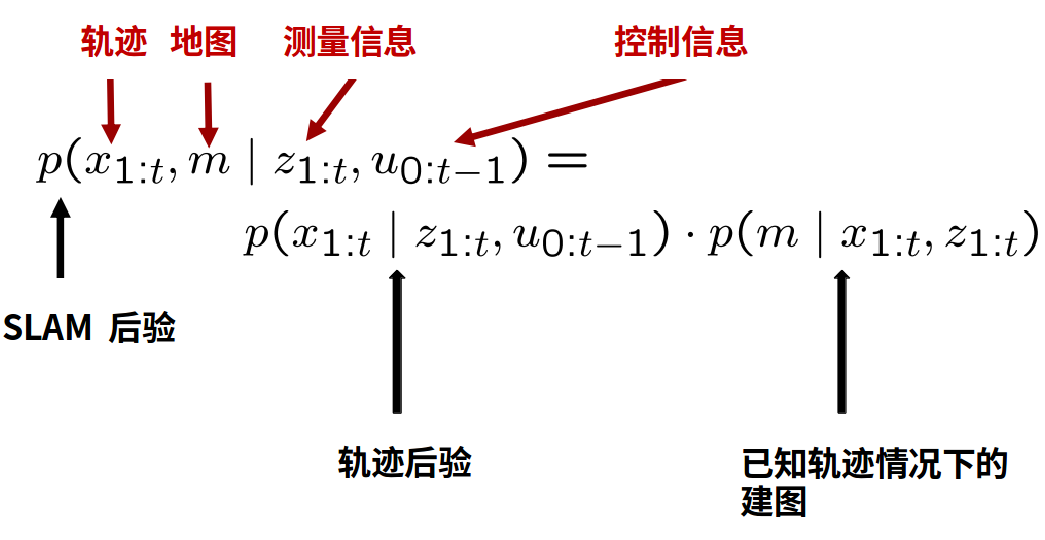图 2. 条件概率

其中建图那一项根据马尔科夫性质省略了 u0:t−1${u}_{0:t-1}$$u_{0:t-1}$ ，因为在已知轨迹和观测信息的情况下，控制信息对于我们的建图而言是没有影响的。这样分解的实际意义就是，先根据测量信息和控制信息计算出机器人的轨迹，然后根据轨迹和测量信息建图。其实目前大部分 SLAM 算法都按照这个思路来实现的，并且主要关注于对运动轨迹的估计和优化上，因为有了精确的轨迹，建图就成了自然而然的事了。

地图的种类

在继续介绍 SLAM 的求解之前，我们先来介绍一下 SLAM 构建的地图的种类。

我所知道的 SLAM 构建的地图一般有两类：volumetric map 和 feature map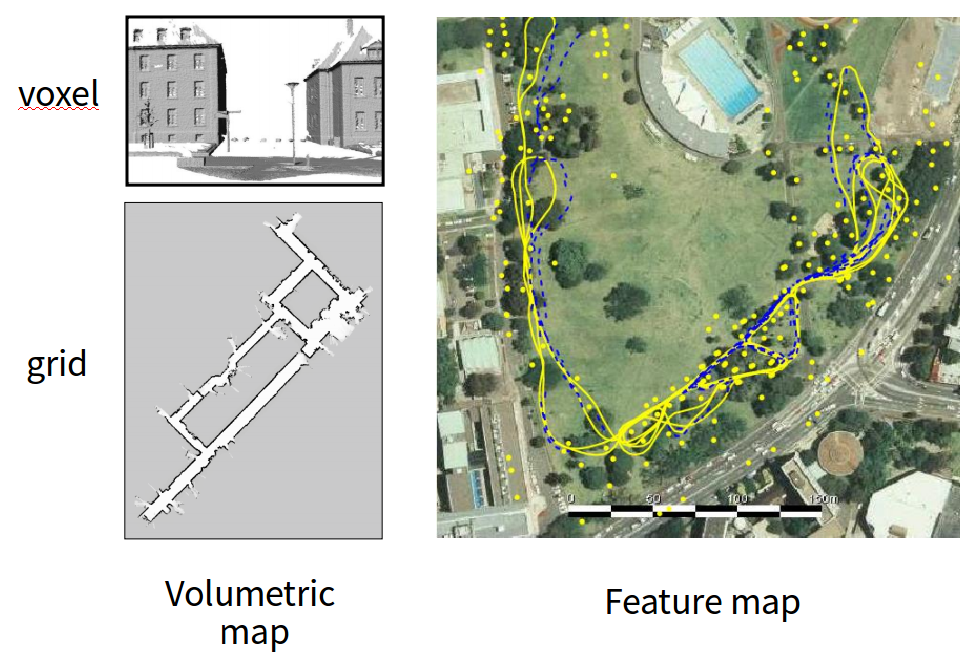图 3. 地图种类

其中 feature map 我没有接触过，我理解的就是在测量信息中提取特征，将这些特征设置为 landmark ，并标示这些 landmark 的位置。这个地图的好处是比较适合动态的场景，因为当 landmark 移动到另一处时，我们只需要改变这个 landmark 的坐标就可以了。

虽然我接触的一直是 volumetric map ，但我还真不知道这该怎么翻译，不过在 2d 中， volumetric map 就是 grid map，也就是栅格地图， 3d 中就是 voxel map，也就是体素地图。因为本文主要讲的是 2d SLAM ，所以我还是主要讲一下栅格地图。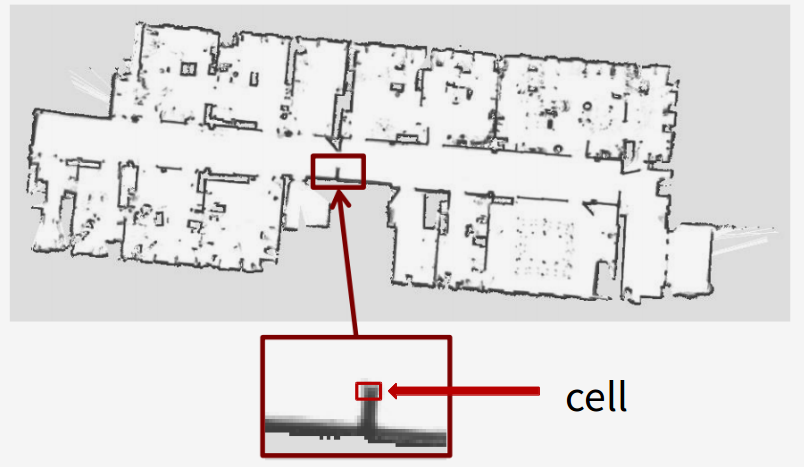图 4. 栅格地图

栅格地图主要就是把地图离散化为一个个的单元(cell)，每个单元占据 r∗r$r\ast r$$r*r$ 个像素，所以 r$r$$r$ 的大小就代表了地图的分辨率，位置在 (xi,yi)$\left({x}_{i},{y}_{i}\right)$$(x_i, y_i)$ 的 cell 的 index 为 i=xi∗y+yi$i={x}_{i}\ast y+{y}_{i}$$i=x_i * y + y_i$。

Assumption：

每个 cell 有两种状态：free、occupied
每个 cell 之间的状态相互独立

当 cell 为 free 时，该 cell 的灰度值就设为 255，也就是白色，当 cell 为 occupied 时，该 cell 的灰度值就设为 0 ，也就是黑色。初始时我们不知道 cell 具体的状态，我们就将其设为 100 ，也就是灰色。

接下来我们设 mi${m}_{i}$$m_i$ 为第 i$i$$i$ 个 cell 是否occupied 的随机变量，那么 p(mi=1)$p\left({m}_{i}=1\right)$$p(m_i=1)$ 或 p(mi)$p\left({m}_{i}\right)$$p(m_i)$ 就表示第 i$i$$i$ 个 cell 为 occupied 的概率，所以 p(mi)=1$p\left({m}_{i}\right)=1$$p(m_i) = 1$ 就表示第 i$i$$i$ 个 cell 为 occupied ，p(mi)=0$p\left({m}_{i}\right)=0$$p(m_i) = 0$ 就表示第 i$i$$i$ 个 cell 为 free ，如下图 5 ：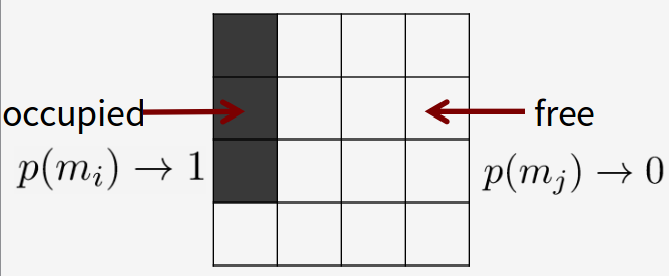图 5. assumption 1

根据 assumption 2 ，我们很容易能得到下式：

p(m)=∏ip(mi)$p\left(m\right)=\prod _{i}p\left({m}_{i}\right)$p(m) = \prod_i p(m_i)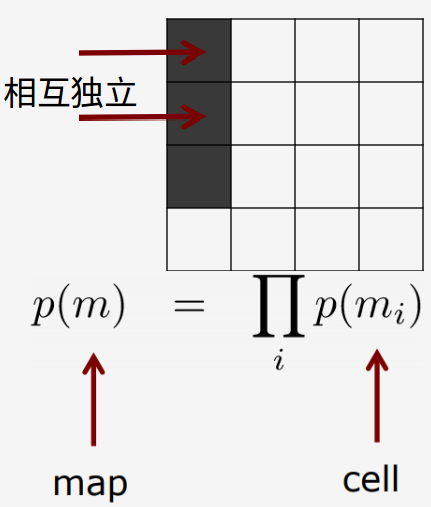图 6. assumption 2

有了这些基础概念，我们接下来就可以介绍各种具体的 SLAM 算法了。

已知轨迹的条件下建图

这里我们先介绍一下如何在已知轨迹的条件下建图。也就是在给定传感器数据 z1:t${z}_{1:t}$$z_{1:t}$ 和轨迹信息 x1:t${x}_{1:t}$$x_{1:t}$ 的条件下估计 t$t$$t$ 时刻的地图 m$m$$m$ ，即 p(m|z1:t,x1:t)$p\left(m|{z}_{1:t},{x}_{1:t}\right)$$p(m|z_{1:t},x_{1:t})$。上节我们说过，各个 cell 之间的状态是相互独立的，所以我们能够得到：

p(m|z1:t,x1:t)=∏ip(mi|z1:t,x1:t)$p\left(m|{z}_{1:t},{x}_{1:t}\right)=\prod _{i}p\left({m}_{i}|{z}_{1:t},{x}_{1:t}\right)$p(m|z_{1:t},x_{1:t}) = \prod_i p(m_i|z_{1:t},x_{1:t})

也就是我们单独的为每个 cell 计算 p(mi|z1:t,x1:t)$p\left({m}_{i}|{z}_{1:t},{x}_{1:t}\right)$$p(m_i|z_{1:t},x_{1:t})$ 即可。这里介绍一种最简单的方法——基于频率的方法——帮助我们快速理解这一计算过程。

首先定义各种用到的各种变量：

mit${m}_{t}^{i}$$m_t^i$：第 i$i$$i$ 个 cell 在 t$t$$t$ 时刻是否被 occupied

vit${v}_{t}^{i}$$v_t^i$：第 i$i$$i$ 个 cell 被观测的次数，也就是被激光扫描到的次数

bit${b}_{t}^{i}$$b_t^i$：观测到第 i$i$$i$ 个 cell 被 occupied 的次数

p(mit)$p\left({m}_{t}^{i}\right)$$p(m^i_t)$ 的计算如下所示

每当接收到一次观测数据时，计算
(bit,vit)={(bit−1+1,vit−1+1)(bit−1,vit−1+1)ifoccupiediffree$\left({b}_{t}^{i},{v}_{t}^{i}\right)=\left\{\begin{array}{cc}\left({b}_{t-1}^{i}+1,{v}_{t-1}^{i}+1\right)& \mathrm{i}\mathrm{f}\phantom{\rule{thickmathspace}{0ex}}\mathrm{o}\mathrm{c}\mathrm{c}\mathrm{u}\mathrm{p}\mathrm{i}\mathrm{e}\mathrm{d}\\ \left({b}_{t-1}^{i},{v}_{t-1}^{i}+1\right)& \mathrm{i}\mathrm{f}\phantom{\rule{thickmathspace}{0ex}}\mathrm{f}\mathrm{r}\mathrm{e}\mathrm{e}\end{array}$(b^i_t,v^i_t)=
\left\{
\begin{array}{cc}
(b^i_{t-1}+1,v^i_{t-1}+1) & \mathrm{if\;occupied}\\
(b^i_{t-1},v^i_{t-1}+1) & \mathrm{if\;free}
\end{array}
\right.

p(mit)=bitvit$p\left({m}_{t}^{i}\right)=\frac{{b}_{t}^{i}}{{v}_{t}^{i}}$p(m^i_t)=\frac{b^i_t}{v^i_t}

基于频率的方法比较简单粗暴，缺点是对 cell 的初始状态考虑不足。

《Probabilistic Robotics》 C9.2 介绍了更通用的算法来计算 p(mi|z1:t,x1:t)$p\left({m}_{i}|{z}_{1:t},{x}_{1:t}\right)$$p(m_i|z_{1:t},x_{1:t})$ ，这里就先不介绍了，书上说的也比较明白。

总之，我们需要知道的就是，在已知运动轨迹的条件下进行建图是比较简单的， SLAM 真正的难点应该是估计机器人的运动轨迹，也可以说是估计某时刻的位置。

已知地图的条件下预测轨迹

这一节介绍一下如何在已知地图的条件下预测机器人的运动轨迹，也就是 p(x1:t|z1:t,u0:t−1)$p\left({x}_{1:t}|{z}_{1:t},{u}_{0:t-1}\right)$$p(x_{1:t}|z_{1:t},u_{0:t-1})$ ，也许你会有个疑问：不是说先预测轨迹，才有地图的么，怎么这里能用地图信息来预测轨迹呢？而且这里的条件概率里也没有以地图信息作为条件啊。

这里我们将要求解的式子稍微改变一下，改为求
p(xt|xt−1,mt−1,zt,ut−1)$p\left({x}_{t}|{x}_{t-1},{m}_{t-1},{z}_{t},{u}_{t-1}\right)$p(x_t|x_{t-1},m_{t-1},z_t,u_{t-1})
也就是在已知 t−1$t-1$$t-1$ 时刻的机器人位置、地图和控制信息，以及 t$t$$t$ 时刻的观测信息的条件下，估计 t$t$$t$ 时刻的机器人位置。其实这个式子跟之前那个是一样的，只不过一个估计的是轨迹，一个估计的是下一时刻的位置，实际上大多数 SLAM 都是迭代式的估计下一时刻的位置来进行建图的。接下来我就介绍一下粒子滤波是如何应用到机器人的位置估计的。

首先我们需要的其实就是能够得到上式的概率密度函数，然后找到使该概率密度函数取最大值时的 xt${x}_{t}$$x_t$，但是这个概率密度函数很难直接求解，于是人们就想到了用蒙特卡洛的方法通过样本来近似这个分布，进而求出 xt${x}_{t}$$x_t$ 。这也就是基于蒙特卡洛的定位算法。

这里先通过图示来大致介绍一下蒙特卡洛方法是如何帮助机器人定位的。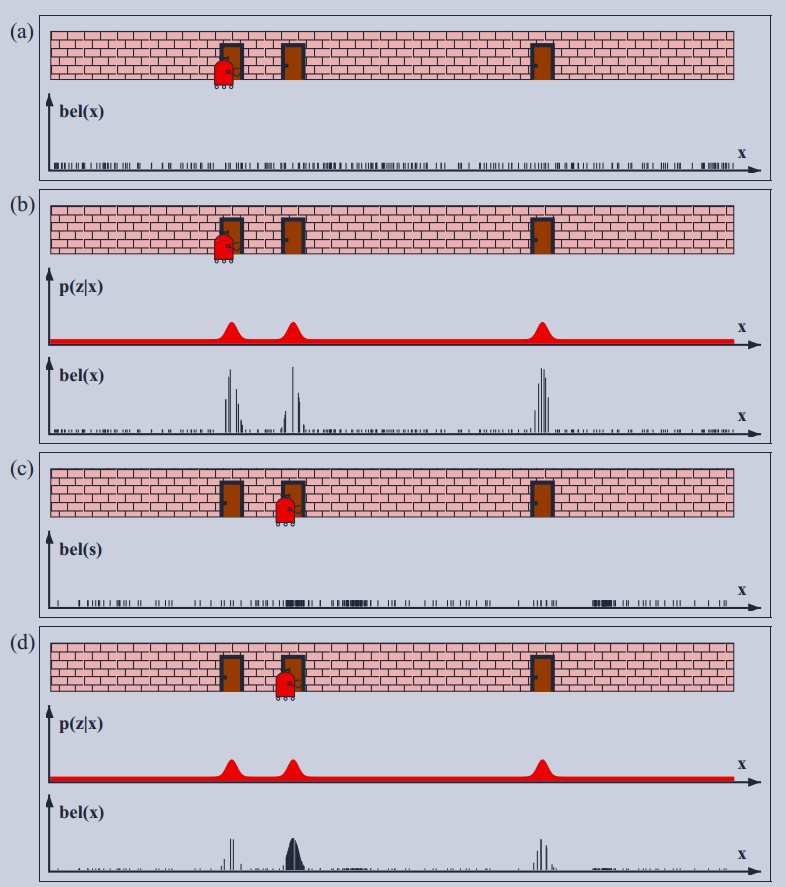图 8. 蒙特卡洛定位

蒙特卡洛方法其实就是通过样本来近似概率分布。如图 8.a ，在没有任何观测数据的时候，机器人完全不知道自己在地图中的什么位置，因此此时它的位置在地图中应该是一个均匀分布，那我们就随机在地图中选取 100 个样本，各个样本的位置如图 8.a 的下图所示。

但好在我们的机器人有“一双眼睛”，可以看到自己目前是在一扇门的前面，那么我们就可以对每个样本计算从该位置看到的门与机器人“眼睛”看到的门的匹配程度了，匹配程度越高，我们也就为该样本赋予更高的权重，如图 8.b 的下图所示。

然后我们根据每个样本的权重对所有样本进行有放回的重新采样，直观上来说，我们应该能想到在三个门附近的样本数会更多一些，其他地方的样本数会比之前均匀分布的要少一些，也就是如图 8.c 的下图所示。不过这里的图 8.c 是机器人往右移动了一段距离后的图像，因此你会看到下图中三个门附近的样本都往右平移了一段距离。

现在机器人又看到自己在一个门的前面了，跟之前的操作一样，我们还是对每个样本计算它在地图中的匹配程度，匹配程度越高，我们就赋予该样本更高的权重，然后根据权重重新采样，不断重复这一过程。移动一段距离之后这些样本就能大致收敛到一处了，机器人也就能大概知道自己在地图中的位置了。

图 9 就是一个采用蒙特卡洛定位的真实例子，图中的黑点是一个样本，也就是一个位置估计，在运动到图 c 的位置时，位置估计就比较准确了。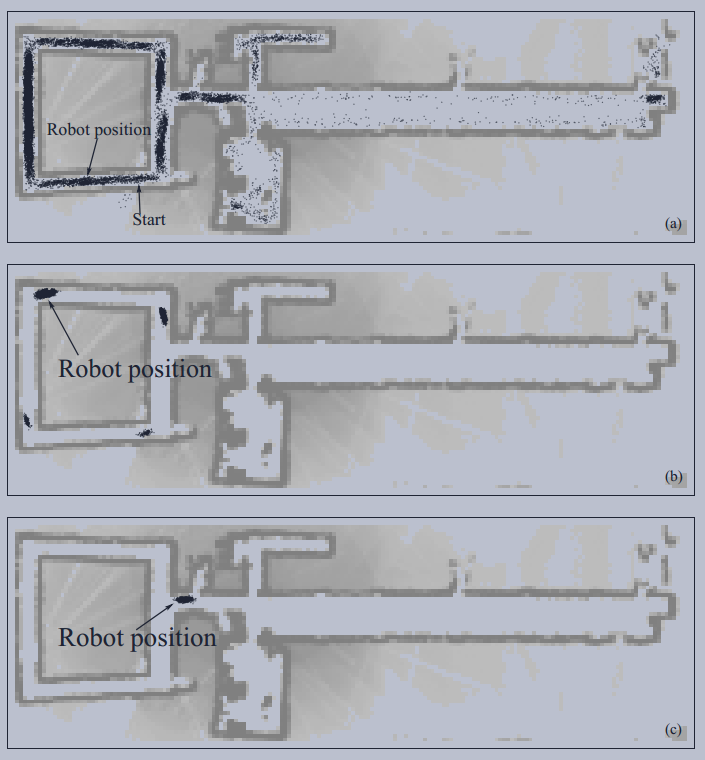图 9. 蒙特卡洛定位实例

这里给出蒙特卡洛定位的算法：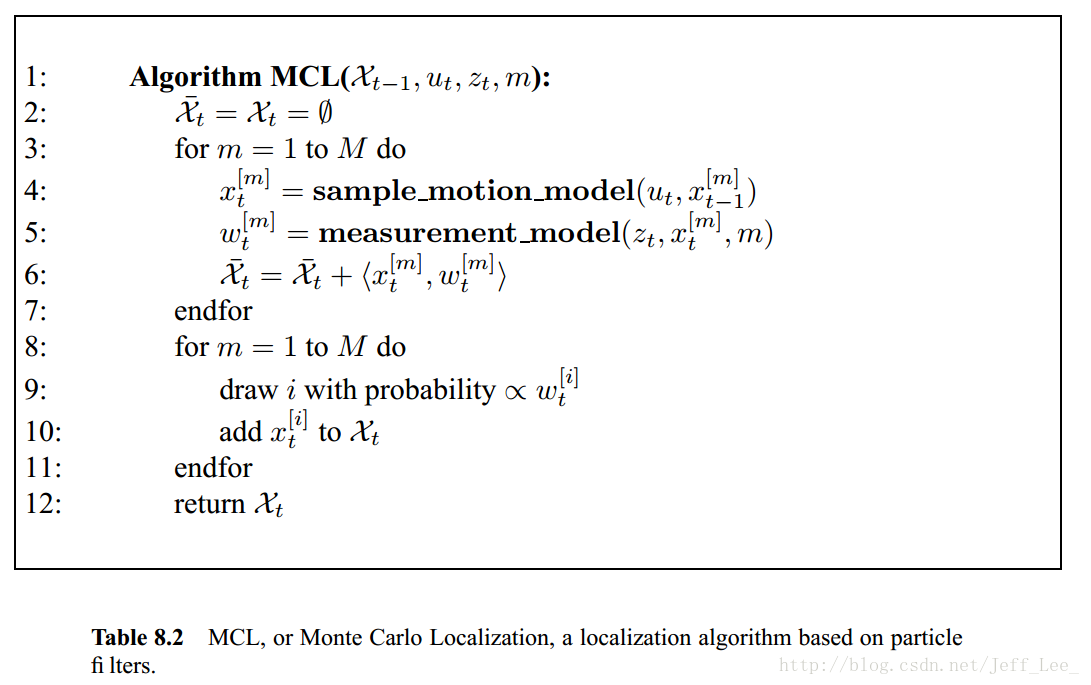图 10. 蒙特卡洛定位算法

这里的 χ$\chi$$\chi$ 就是所有的样本集，算法背后的数学原理如图 11 所示。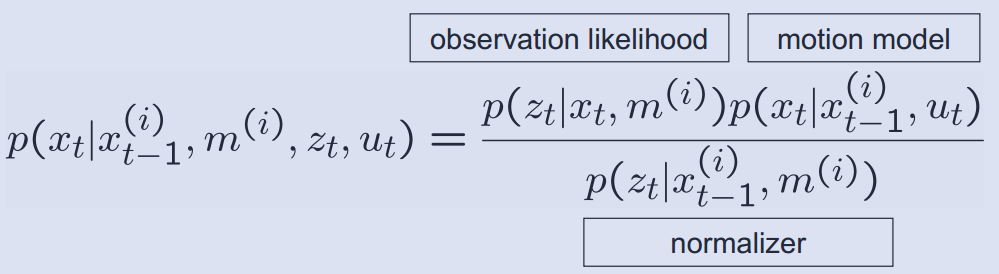图 11. 贝叶斯分解

其中 measurement_model 就是计算图 11 中 observation likelihood，算法中没有计算 normalizer 的部分，这是因为每个样本的权重会标准化为 Σwi=1$\mathrm{\Sigma }{w}_{i}=1$$\Sigma w_i = 1$ ，而 normalizer 与 xt${x}_{t}$$x_t$ 无关，所以在标准化时会被抵消掉，不需要计算。

参考资料

Probabilistic Robotics. S.Thrun

grid-maps：http://ais.informatik.uni-freiburg.de/teaching/ws15/mapping/pdf/slam10-gridmaps.pdf

RBPF：http://webdiis.unizar.es/~neira/5007439/stachniss-rbpfmapping.pdf
展开全文• 用于低纹理和动态环境的IMU辅助2D SLAM方法研究论文
• % 2D SLAM with linear KF - Moving vehicle - Relative measurement - % Unlimited Sensor Range - Observing (x,v,landmarks) % % A 2 DOF mobile robot is moving along a path detecting some % motionless ...
% 2D SLAM with linear KF - Moving vehicle - Relative measurement -
% Unlimited Sensor Range - Observing (x,v,landmarks)
%
% A 2 DOF mobile robot is moving along a path detecting some
% motionless landmarks; the position/velocity of the robot and position
% of landmarks are computed by means of Simultaneos Localization and
% Mapping using a linear Kalman Filter.
% All the measures are relative to robot position.
% The measuring range sensor is unlimited.
%
% Programmed by Joaquim Salvi

clear all;
NumberTimeStamps = 2000;
MapDimension = [1,100;1,100];

% INTRODUCE LANDMARKS
figure(1); clf; title('Introduce Landmarks');
v=[MapDimension(1,1) MapDimension(1,2) MapDimension(2,1) MapDimension(2,2)];
axis(v); hold on;
pin=1; button = 0;
nlandmarks=0;
% Get input landmarks graphically, click right button to finish
while button ~= 3
[x,y,button]=ginput(1);
pin= ~isempty(x);
if pin && button ~= 3
nlandmarks = nlandmarks+1;
plot(x,y,'r*')
pp(:,nlandmarks)=[x;y];
end
end
hold off;
NumberLandmarks = size(pp,2);

% DEFINE TRAJECTORY
% Definition of the real trajectory; vehicle with constant velocity
figure(2); clf;
title('Introduce trajectory around landmarks');
v=[MapDimension(1,1) MapDimension(1,2) MapDimension(2,1) MapDimension(2,2)];
axis(v); hold on;
plot(pp(1,:),pp(2,:),'r*');
pin = 1; button = 0; fi = 0;
npoints = 0; dist = 0;
% get trajectory segments, click right button to finish
while button ~= 3
[x,y,button]=ginput(1);
pin= ~isempty(x);
if pin && button ~= 3
npoints = npoints + 1;
t(:,npoints)=[x;y];
plot(x,y,'go');
if npoints > 1
plot(t(1,npoints-1:npoints),t(2,npoints-1:npoints),'g-');
dist = dist + norm(t(:,npoints) - t(:,npoints-1));
end
end
end
% Sampling NumberTimeStamps points in the given trajectory.
point = 2; dist2=0; incdist=dist/NumberTimeStamps;
tt(:,1)=t(:,1);
for i = 2:NumberTimeStamps
tt(:,i)=tt(:,i-1)+ incdist*((t(:,point)-t(:,point-1))/norm(t(:,point)-t(:,point-1))); % tx,ty trajectories
vv(:,i-1)=tt(:,i)-tt(:,i-1); % vx,vy velocities
plot(tt(1,i),tt(2,i),'b.');
dist2 = dist2 + incdist;
if (dist2 + incdist) > norm(t(:,point)-t(:,point-1)) && abs((dist2 + incdist)-norm(t(:,point)-t(:,point-1))) > abs(dist2-norm(t(:,point)-t(:,point-1)))
point = point + 1; dist2 = 0;
end
end
plot(tt(1,:),tt(2,:),'b.');
hold off;

% DEFINE INITIAL PARAMETERS
v = vv;  % Velocity norm is constant and equal to 1, but not vx, vy
x = tt;  % Trajectory is the interpolated trajectory introduced by user
% Landmarks are arranged in a vector form (x1,y1,x2,y2,... yn)
clear vv;
for i=1:nlandmarks
p(2*i-1)=pp(1,i);
p(2*i)=pp(2,i);
end

% Definition of process and measurement noises
r = (30*0.5)^2;  % landmark measurement noise, change to see effect
rx = (3*0.5)^2; % position measurement noise, change to see effect
rv = (0.1*0.5)^2; % velocity measurement noise, change to see effect
pn = (0.001)^2; % process noise, change to see effect

% Definition of Measurement matrix
% Landmark distance are relative to robot position
H = [diag(ones(2+2,1)) zeros(2+2,2*NumberLandmarks)];
for i=1:2:2*NumberLandmarks
H = [H;-1 0 0 0 zeros(1,i-1) 1 zeros(1,2*NumberLandmarks-i)];
H = [H;0 -1 0 0 zeros(1,i) 1 zeros(1,2*NumberLandmarks-i-1)];
end

% Definition of the State matrix
% The State matrix is a diagonal of ones, which means that the prediction
% of next state is equivalent to current state, except the position which
% is updated with the current velocity at every time stamp.
Fk = diag(ones(1,2+2+2*NumberLandmarks)); Fk(1,3)=1; Fk(2,4)=1;

% Definition of the Control vector
% The control vector is null, which means that the are no external inputs
% that change the robot state.
Uk = zeros(2+2+2*NumberLandmarks,1);

% Definition of the Covariance matrix
% The initial covariance matrix is defined with a large diagonal uncertainity
% in both state of the robot and position of the landmarks and with an
% equivalent uncertainity which means that none prevail over the other.
Pk = diag(ones(1,2+2+2*NumberLandmarks)); Pki=[];
for i = 1:2+NumberLandmarks
Pki = [Pki [1 0;0 1]];
end
Pk(1:2,:) = Pki;
Pk(:,1:2) = Pki';
Pk = r*Pk;
Pk(1,1)=rx+rv; Pk(2,2)=rx+rv; Pk(3,3)=rv; Pk(4,4)=rv;
Pk(1,3)=rv; Pk(2,4)=rv; Pk(3,1)=rv; Pk(4,2)=rv;
for i = 5:5+2*NumberLandmarks-1
Pk(i,i) = r+rx;
end

%Definition of the Process/Measure variance; landmarks are motionless so
%they are not influenced by noise.
Q = diag([pn*ones(1,2+2) 0*pn*ones(1,2*NumberLandmarks)]);
%Definition of the Measurement Noise Matrix
R = diag([rx*ones(1,2) rv*ones(1,2) r*ones(1,2*NumberLandmarks)]);

% Initial prediction of the vector state
xhat(:,1)= [x(:,1)' v(:,1)' p]';
N = sqrt(pn)*randn(2+2,1); xhat(1:4,1) = xhat(1:4,1) + N; % adding process noise

% KALMAN FILTER LOOP
figure(3); clf;
handle = gcf;
set(handle,'DoubleBuffer','on')

for k = 1:NumberTimeStamps-2

% Prediction
xhat(:,k+1)=Fk*xhat(:,k)+Uk;     % Prediction of next state
zhat(:,k+1)= H*xhat(:,k+1);      % Measure at the predicted position
Pk=Fk*Pk*Fk' + Q;

% Observation
N = [sqrt(rx)*randn(2,1); sqrt(rv)*randn(2,1); sqrt(r)*randn(2*NumberLandmarks,1)];
z(:,k+1)= H*[x(:,k+1)' v(:,k+1)' p]' + N; % Measure at the correct position x(k+1) v(k+1) and p
vv(:,k+1)=z(:,k+1)-zhat(:,k+1);  % Innovation vector, i.e. discrepancy between measures
S = H*Pk*H' + R;

% Update
W = Pk * H' * inv(S);
xhat(:,k+1)=xhat(:,k+1)+W*vv(:,k+1);
Pk = Pk - W*H*Pk;
Un(:,k+1)=diag(Pk);  % The Uncertainity is stored for plotting purposes

% Plotting results
clf; hold on;
title('2D SLAM with KF');
mapdim=[MapDimension(1,1) MapDimension(1,2) MapDimension(2,1) MapDimension(2,2)];
axis(mapdim);
plot(x(1,1:k),x(2,1:k),'g');
plot(xhat(1,1:k),xhat(2,1:k),'r');
plot(x(1,k+1),x(2,k+1),'g.');
plot(xhat(1,k+1),xhat(2,k+1),'r.');
[xe,ye,ze]=ellipsoid(xhat(1,k+1),xhat(2,k+1),0,3*sqrt(Un(1,k+1)),3*sqrt(Un(2,k+1)),0);
plot(xe(floor(size(xe,2)/2),:),ye(floor(size(ye,2)/2),:));
plot(pp(1,:),pp(2,:),'r*');
for j=5:2:5+2*NumberLandmarks-1
%        plot(xhat(1,1:k+1)+zhat(j,1:k+1),xhat(2,1:k+1)+zhat(j+1,1:k+1),'m.');
plot(xhat(j,k+1),xhat(j+1,k+1),'b.');
[xe,ye,ze]=ellipsoid(xhat(j,k+1),xhat(j+1,k+1),0,3*sqrt(Un(j,k+1)),3*sqrt(Un(j+1,k+1)),0);
plot(xe(floor(size(xe,2)/2),:),ye(floor(size(ye,2)/2),:));
end
drawnow;
hold off;

end;

% PLOTTING RESULTS
figure(4); clf; title('2D SLAM with KF');
mapdim=[MapDimension(1,1) MapDimension(1,2) MapDimension(2,1) MapDimension(2,2)];
axis(mapdim); hold on;
for i = 1:NumberTimeStamps-2
plot(x(1,i),x(2,i),'g.');
plot(xhat(1,i),xhat(2,i),'r.');
[xe,ye,ze]=ellipsoid(xhat(1,i),xhat(2,i),0,2*sqrt(Un(1,i)),2*sqrt(Un(2,i)),0);
plot(xe(floor(size(xe,2)/2),:),ye(floor(size(ye,2)/2),:));
for j=5:2:5+2*NumberLandmarks-1
plot(xhat(1,i)+zhat(j,i),xhat(2,i)+zhat(j+1,i),'m.');
plot(xhat(j,i),xhat(j+1,i),'b.');
if mod(i,10) == 2
[xe,ye,ze]=ellipsoid(xhat(j,i),xhat(j+1,i),0,2*sqrt(Un(j,i)),2*sqrt(Un(j+1,i)),0);
plot(xe(floor(size(xe,2)/2),:),ye(floor(size(ye,2)/2),:));
end
end
end
plot(pp(1,:),pp(2,:),'r*');
for j=5:2:5+2*NumberLandmarks-1
plot(xhat(j,NumberTimeStamps-2),xhat(j+1,NumberTimeStamps-2),'go');
end
hold off; zoom on;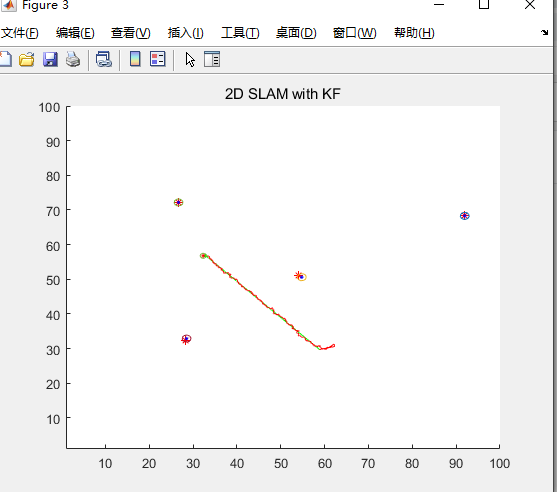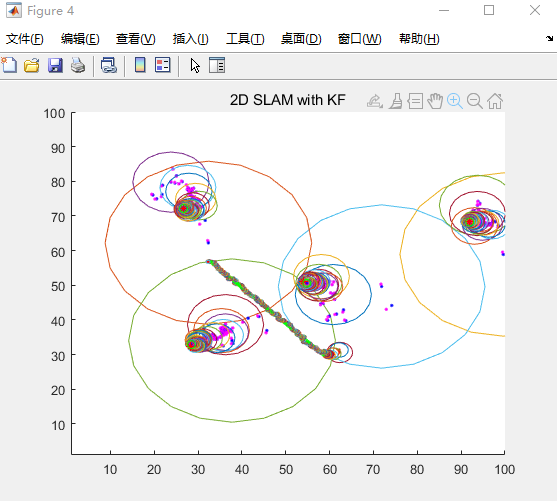D60

展开全文• s 2D SLAM and met the problem that the wall, which once has been recognized, be disappeared when the robot returns. <p>It had not been happend with Gmapping, so I want the cartographer's result to...
• I am trying to perform 2d SLAM using cartographer_ros with Scanse sweep lidar (2d). I have installed the sweep_ros and this allowed me to get point cloud from Scanse sweep lidar and view it. Then I ...
• 初学2D SLAM从无到有1.将/scan数据转化为PointCloud数据2.将/odom数据在Matlab图像显示3.将两个坐标系的坐标变换发布到新的topic进行并进行保存4.采用/odom与/pointcloud以及它们之间的坐标变换进行粗建图5.对每一帧...
1.将/scan数据转化为PointCloud数据.
2.将两个坐标系的坐标变换发布到新的topic进行并进行保存.
3.采用/odom与/pointcloud以及它们之间的坐标变换进行粗建图
4.对每一帧数据进行线段特征提取
未完待续……
可以与我共同探讨lizhouliao@cqu.edu.cn


展开全文自动驾驶
• 自己动手写全套无人驾驶算法系列（一）机器人2D SLAM 一、概述 1.1 系列整体概述： 本文主要介绍无人驾驶SLAM各个环节的各个算法，主要包括4个方面：传感器层，建图层，匹配层和融合定位层。运动模型为差分驱动模型...
自己动手写全套无人驾驶算法系列（四）机器人2D SLAM

目录:
一、概述
1.1 系列整体概述
二、传感器层
2.1 轮式里程计
2.2 IMU
2.3 激光雷达
2.4 视觉VO
三、建图层
3.1 静态二值贝叶斯滤波
3.2 Ray casting算法
3.3 Beam end model算法
3.4 Likelihood range finder算法
3.5 多分辨率地图及内存管理问题
3.5.1 ROS存储
3.5.2 稀疏存储
3.5.3 块存储
3.5.4 直接点云存储
四、匹配算法
4.1 Brute force（含分数计算的三种方法）
4.2 Compute 2D slice
4.3 Multiple resolution match
4.4 Fast scanmatching
4.5 ICP
4.6 NDT
4.7 NDT+ICP
4.8 插值匹配
4.9 总结
五、前端融合算法
5.1 EKF
5.2 比例融合
六、后端融合算法
6.1 EKF
6.2 UKF
6.3 PF
6.4 Graph Optimizer
一、概述
1.1 系列整体概述：
本文主要介绍无人驾驶SLAM各个环节的各个算法，主要包括4个方面：传感器层，建图层，匹配层和融合定位层。运动模型为差分驱动模型，目前代码已经做完，但因为要对其专利保护，日后再放出，特此先作介绍，并提供交流。
欢迎大家对我的github项目提出BUG，或者需要我更新新的算法,或者需要技术合作，都可以联系我：
163邮箱：wujiazheng2020@163.com
gmail:wujiazheng2020@gmail.com
QQ群：710805413
二、传感器层
2.1 轮式里程计
轮式里程计的来源主要是编码器或者CAN传入PC端的小车的轮子速度和加速度信息，我们通过轮子的速度积分可以得到车辆已经行走的坐标信息，或者编码器也可以直接提供小车已经行走的坐标信息，同时我们也可以得到车辆的速度信息，将其可以转换为twist，即成了估计车辆位姿的重要来源。但是我们要注意，因为时间的延迟，我们必须将时间对齐固定时钟源，比如我的代码里对齐的是激光雷达的时间，如果轮式早到则要按运动学模型推到相应时间，同时按v及w可以得到相应写方差矩阵
2.2 IMU
imu可以提供3个轴的旋转信息和3个方向的加速度信息，加速度积分两次可以得到位移，但一般考虑精度问题不这么做，除非你的IMU是上千上万那种可以考虑这么做，我们主要从IMU得到旋转速度及旋转角度两个数据，同时按IMU误差模型可以得到其协方差矩阵，可见IMU误差模型。同样的，imu也需要时间对齐。
2.3 激光雷达
激光雷达信息往往是一个角度+一个距离，我们通过距离×cos（角度）或sin(角度)既可以把每个激光束所表示的点的坐标算出来，又因为运动的原因，比如激光雷达的时间间隔是0.2s，一共360个点，那么第1个点就需要做0.2/360 s的运动畸变矫正，我们知道车辆的速度和角速度，即可通过运动模型矫正激光点云，其他点也是按同样的方法矫正。如下：
for(U4 i = 0;i<scan_t.size();i++){
yaw = scan_t[i].th;
if(yaw < 0){
yaw += 2*TT;
}
yaw_res = end_angle - yaw;
time_res = lidar_intv*(yaw_res/all_angle);
wdt = wt*time_res;
scan_t[i].x -= ( vbw*sin(wheel_th) + vbw*sin(wheel_th+wdt) );
scan_t[i].y += ( vbw*cos(wheel_th) - vbw*cos(wheel_th+wdt) );
}

而vw的获得主要有两种方法：
1.轮式里程计及IMU辅助
2.雷达两桢速度不变假设。
2.4 视觉VO
本人的代码尚未提及，对于扫地机器人等2d小型机器人，直接激光雷达方案，或者视觉VIO以MSCKF都可以，这属于单独的内容了，故不作提及。
三、建图层
本人的代码主要提供3大见图算法，都用到了bresenhamline，其实就是电脑中画直线的算法，具体可见bresenham算法，其过程一般是先用建图算法建图再用静态二值贝叶斯滤波融合。如下：
3.1 静态二值贝叶斯滤波
ROS中将全局地图与局部地图对齐后如果，全局地图值为-1（未探测），即把局部地图的值直接赋过去即可，但若全局地图有值，那么则要进行静态二值贝叶斯滤波：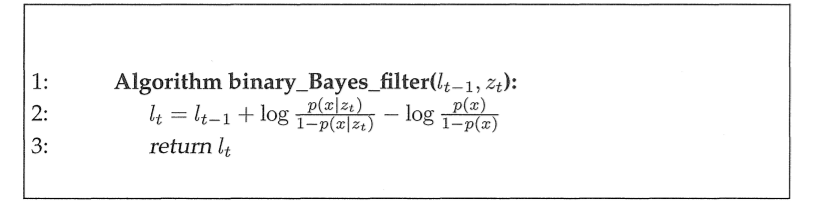P(x)就是原来的全局地图对应栅格概率0-1，P(X|Zt)就是局部地图对应栅格概率，L0就是全局为未探测时候覆盖的局部地图值，之后一步步更新就可了。效果如下图：(让局部地图向着右上不断移动，并扩张和融合到全局地图)。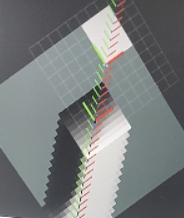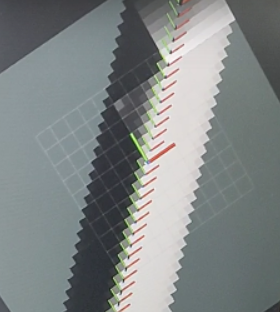3.2 Ray casting算法
对每个激光雷达点转换到局部地图坐标系后直接使用bresenham探测，在击中点附近半径小于hit_r的范围概率置为0.95,（ROS值是95,扩大了100倍节省内存）,其他点都置为0.01即可。每次见图完用静态二值贝叶斯滤波融合即可，如下：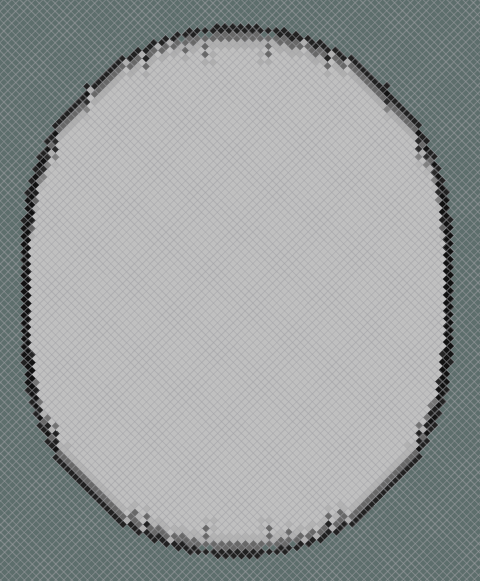3.3 Beam end model算法
波束模型，使用前我们要用EM算法算出激光雷达的Zhit,Zshort,Zmax,Zrand及hit的方差和均值，short分布的lambda：如下：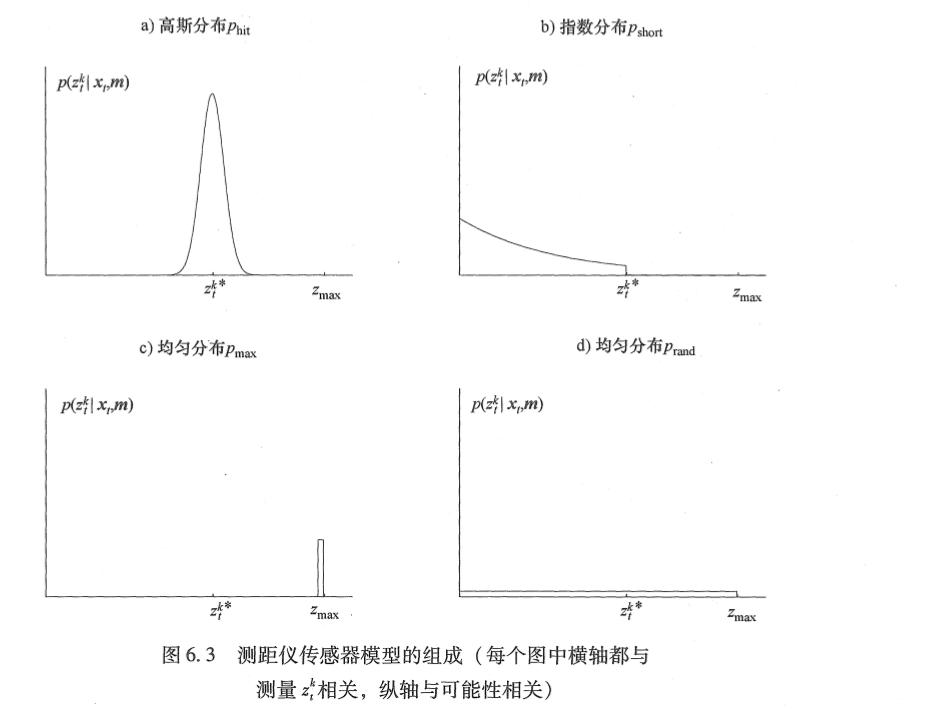以如下代码：
void Mapper::Static_EM_Estimation_For_Z(std::vector<R8> z_m,std::vector<R8> z_r,Mapper_Param &param){
U4 z_size = z_m.size(); // the size of z_m and z_r must be equal
if(z_r.size() != z_size || z_size == 0){
return ;
}
R8 z_hit   = param.z_hit;
R8 z_short = param.z_short;
R8 z_max   = param.z_max;
R8 z_rand  = param.z_rand;
R8 z_hit_abs = z_hit*z_size;
R8 z_short_abs = z_short*z_size;
R8 sigma2 = 0,lamda = 0;
for(U4 i = 0; i< z_size; i++){
sigma2 += (z_m[i] - z_r[i])*(z_m[i] - z_r[i]);
lamda  += z_m[i];
}
sigma2 = sigma2/z_hit_abs;
lamda = z_short_abs/lamda;
R8 Eta = 0;
R8 pre_sigma2 = 0,pre_lamda = 0;
R8 p_hit_i,p_short_i,p_max_i,p_rand_i,b2;
R8 e_hit_i,e_short_i,e_max_i,e_rand_i;
R8 abs_z,e_hit_all,e_short_all,e_max_all,e_rand_all;
R8 e_hit_sqr_all,e_short_z_all;
p_max_i = 0;
p_rand_i = param.min_rslo/param.range_max;

U4 times = 0;
while(fabs(pre_sigma2 - sigma2) >= 1e-3 || fabs(pre_lamda - lamda) >= 1e-3){
abs_z = 0,e_hit_all = 0,e_short_all = 0,e_max_all = 0,e_rand_all = 0;
e_hit_sqr_all = 0,e_short_z_all = 0;
pre_sigma2 = sigma2;
pre_lamda  = lamda;
for(U4 i = 0; i<z_size;i++){
b2 = (z_m[i] - z_r[i])*(z_m[i] - z_r[i])/(2*sigma2);
p_hit_i = exp(-b2)/(2*TT*sigma2);
p_short_i = lamda*exp(-lamda*z_m[i]);
Eta = 1/(p_hit_i + p_short_i + p_max_i + p_rand_i);
e_hit_i = Eta*p_hit_i;
e_short_i = Eta*p_short_i;
e_max_i = Eta*p_max_i;
e_rand_i = Eta*p_rand_i;

abs_z += (e_hit_i + e_short_i + e_max_i + e_rand_i);
e_hit_all += e_hit_i;
e_short_all += e_short_i;
e_rand_all += e_rand_i;
e_hit_sqr_all += (e_hit_i*(z_m[i]-z_r[i])*(z_m[i]-z_r[i]));
e_short_z_all += (e_short_i*z_m[i]);
}
z_hit   = e_hit_all/(abs_z);
z_short = e_short_all/(abs_z);
z_max   = 0;
z_rand  = e_rand_all/(abs_z);
sigma2  = e_hit_sqr_all/e_hit_all;
lamda   = e_short_all/e_short_z_all;
times ++;
if(times >= 100){
break;
}
}

param.z_hit    = z_hit;
param.z_short  = z_short;
param.z_max    = z_max;
param.z_rand   = z_rand;
param.sigma2   = sigma2;
param.lambda   = lamda;
}

最后需要融合各个分布到如下分布：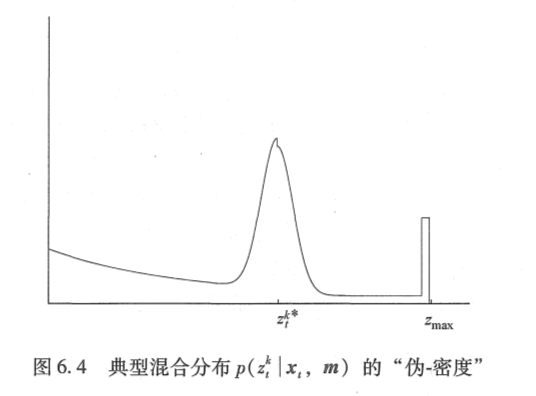然后在bresenham line 上根据离终点和起点的距离算出其概率填入局部地图即可。如下：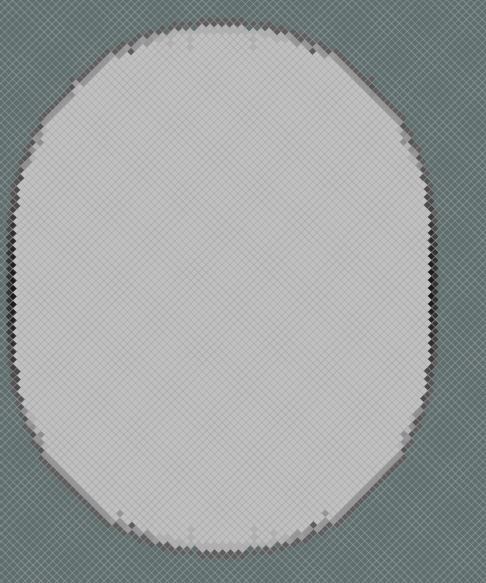其中数值积分按如下代码：
    R8 Mapper::Integral_From_Function(X1 mode,R8 start,R8 end,Mapper_Param &param){
R8 result=0,u,sigma2,lambda;
R8 interval = param.intg_intv;
if(mode != Gaussian && mode != Exp){
return 0;
}
if(mode == Gaussian){
u = param.u;
sigma2 = param.sigma2;
for(R8 i = start;i<end;){
result += 1/sqrt(2*TT*sigma2)*exp(-(i-u)*(i-u)/(2*sigma2))*interval;
i += interval;
}
}
if(mode == Exp){
lambda = param.lambda;
start = start<0? 0:start;
result = exp(-lambda*start)-exp(-lambda*end);
}
return result;
}


这里有个小技巧，当激光雷达不是很准的时候，主要依赖图优化去优化，这时就可以把最高概率的点设置为Pmax比如95,其他概率按最高概率按比例放缩，这样的图更具有信息，不容易被静态二值滤掉。
3.4 Likelihood range finder算法
说白了在bresenham line上每个点，以波束模型算出的那个分布取Phit那个分布，用kdtree搜索其离最近点的距离，代入分布中即得到概率，得到局部地图同样用SBF融合即可，容易出现穿墙问题，是gmapping的建图算法,如下：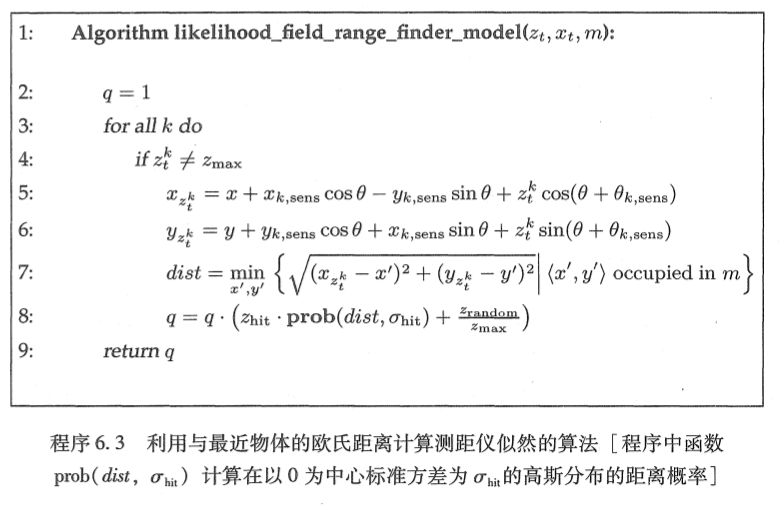核心是使用如下pcl结构：
 pcl::PointCloud<pcl::PointXY>::Ptr cloud (new pcl::PointCloud<pcl::PointXY>);
pcl::KdTreeFLANN<pcl::PointXY> kdtree;

效果如下：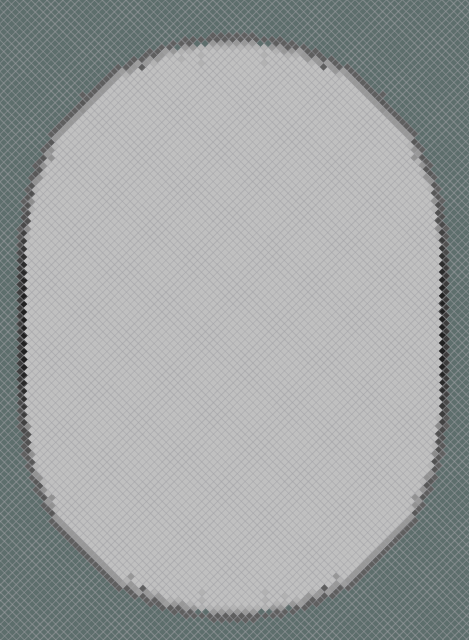3.5 多分辨率地图及内存管理问题
建图的时候需要在多分辨率建图，这时就需要考虑内存问题，少内存必然带来的是低速度，所以必须就内存和速度进行权衡，权衡是按如下公式计算，
假设电脑内存为M,而一个地图点是1字节，故地图边长最大栅格数G_length = sqrt(M)，设地图最小分辨率为rslo，那么地图最大边长L为G_length*rslo, 多分辨率下，其等比数列收敛于1/4，即最大边长为L/(5/4),如果你内存不够就要用磁盘，现在固态的速度300MB-2000MB/s，速度其实是够的，如果是机械就比较麻烦。
3.5.1 ROS存储
存储方式可以用ROS这种，就是一维数组，x = i%width y = i/width;
3.5.2 稀疏存储
也可以是稀疏存储，当地图填充率小于1/9的时候即使用，结构体如下：
class Map_Data{
I4 x;
I4 y;
X1 val;
}

3.5.3 块存储
比较常用，就是分割地图为一个个正方形块，可以存储到磁盘中，使用的时候按当前归化坐标索引即可，花费只是索引时间，存储也是一样，最多的时候只需取4块即可，保存的时候文件的名字可以帮助你快速索引，并且排序由系统命令完成即可。
文件名如下:   5_10.ff 我用了不同文件夹放不同分辨率的地图，所以每个文件直接以栅格坐标xy命名即可。而地图边长这些都是在程序定义好的，不需要再读取。
3.5.4 直接点云存储
把点云经过VOXEL filter后按时间顺序直接存下来，对于图优化的算法，这种可以在图优化回环结束后再快速建图，故如果你用图优化算法，就用这种存储是可以的，当然也可以加块算法，结构如下：
std::vector<std::vector<Pose_2d>> scan_set;

四、匹配算法
主要有8大算法，其他衍生算法我也会提到。
4.1、Brute force
说白就是暴力匹配，就是在当前位置附近取abc个位置探索分数最高的一个，然后把位置更新过去即可，a是x窗口大小，b是y窗口大小，c是yaw的窗口大小，很耗内存，其中分数的计算方法有两种：1.相关性测量模型，2.插值模型：
相关性测量模型如下：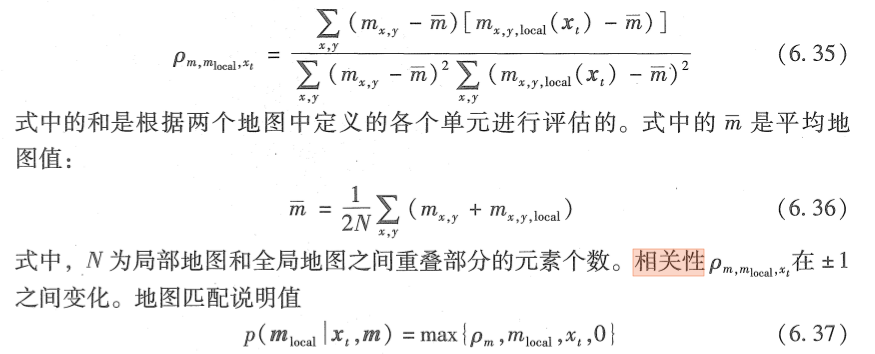即协方差的算法，很简单。
插值模型分为双线性插值和双三次插值：
双线性插值如下：双线性插值，这是hector slam用的插值匹配方法。本用于图像缩放。
双三次插值如下：双三次插值，这是cartographer的插值匹配方法。本用于图像缩放。
然后我们就获得了那个分数。相关性和插值的函数实现如下：
 R8 Get_Score(nav_msgs::OccupancyGrid &local_map,nav_msgs::OccupancyGrid &global_map,X1 mode);
R8 Get_Score(Pose_2d &now_pose,std::vector<Pose_2d> &scan,nav_msgs::OccupancyGrid &global_map,X1 mode);

注：gmapping和cartographer在分数上还加入了离初始点的距离作为分数项，越近分数越高。我的代码沿用这种做法
4.2、Compute 2D slice
说白就是相关性模型你算分数要先算出局部地图，xy的变化可以通过变换地图的起点坐标即可，而angle那个要重新建图，那我直接提前算好不同角度的地图不就可以了。如下：
 for(R8 a = -angle_s;a<=angle_s;a+=angle_inc){
tmp_pose.th = pred_pose.th + a;
map_maker.Get_Local_Map(tmp_pose,scan,param.map_param);
//3.move map and search
for(R8 y = -length_y/2;y <= length_y/2;y+=rslo){
for(R8 x = -length_x/2;x <= length_x/2;x+=rslo){
//待上传后
}
}
}

4.3、Multiple resolution match
可见hector slam，这本是hector slam的算法，先在低分辨率匹配得到粗略的结果再在高分辨率匹配，结果是次优而非最优。其匹配用的是我后面要说的插值匹配。
4.4、Fast scanmatching
这是cartographer的匹配算法，cartographer主要有4种匹配算法，CSM，real time CSM，ceres scanmatching（用ceres做的插值匹配），Fast match（Branch and bound）。real time CSM那个类似compute 2D slice，主要就是branch and bound，说白了粗分辨率的分数要高于细分辨率的分数，因为粗分辨率类似最大采样，细分辨率上打在概率为0上的点，粗分率上可能就是打在障碍物的栅格上，即使是在栅格边缘。有了这个理解，我们先对当前层结点分数算出来排序，取最大的按DFS去搜索，对于叶子节点取最大的一个分数，与我们之前存储的best score比较（初始化为-1），如果大于他就把best node更新为这个叶子节点，回到上一层，如果上一层的老二比这个best score还低，就不进入DFS了，直接再退一层，就按这种递归方式就加速了暴力匹配的速度。参考函数如下：
//2.4 branch and bound like cartographer
Tree_node best_node;
void Branch_And_Brand_DFS(Pose_2d &pred_pose, std::vector<Pose_2d> &scan,Matcher_Param &param,
std::vector<nav_msgs::OccupancyGrid> &multi_map);
Pose_2d Fast_CSM_Matcher(Pose_2d &pred_pose, std::vector<Pose_2d> &scan,Matcher_Param &param,
std::vector<nav_msgs::OccupancyGrid> &multi_map);

4.5、ICP
就是迭代最近点，这个经典算法听的太多了，具体见SLAM十四讲，我是用PCL实现，唯一要注意的就是存储上个点的分配方式：
std::vector<pcl::PointXYZ,Eigen::aligned_allocator<pcl::PointXYZ>> last_scan;

注：实际用的时候必须加入RANSAC，不然难以得到很好的效果。
4.6、NDT
正态分布变换，可见正态分布变换，类似插值匹配，不过是用高斯分布模型。常用于粗匹配与ICP结合使用。
4.7、NDT+ICP
先NDT得到一个粗略的值，再ICP，参考如下：
Pose_2d Matcher::NDT_MIX_ICP_Matcher(Pose_2d &now_pose,Pose_2d &pred_pose, std::vector<Pose_2d> &scan,Matcher_Param &param){
if(first_match){
last_scan = Scan_To_Point(scan);
return pred_pose;
} else {
Pose_2d tmp_pose = NDT_Matcher(now_pose,pred_pose,scan,param);
return ICP_Matcher(now_pose,tmp_pose,scan,param);
}
}

4.8、插值匹配
主要有两种算法：1.线性插值匹配（hector slam） 2.双三次插值匹配（cartographer）。
其形式都类似hectorslam。
其中双三次插值的插值函数和微分函数如下：
R8 Matcher::BiCubic(R8 x,R8 a){
R8 abs_x = fabs(x);
R8 x2 = abs_x*abs_x;
R8 x3 = x2*abs_x;
if(abs_x <= 1){
return (a+2)*x3 - (a+3)*x2 + 1;
}else if(abs_x < 2 && abs_x >1){
return a*x3 - 5*a*x2 + 8*a*abs_x - 4*a;
}else{
return 0;
}
}

R8 Matcher::D_BiCubic(R8 x,R8 a){
R8 x2 = x*x;
if(x > -2 && x < -1){
return -3*a*x2 - 10*a*x - 8*a;
} else if(x >= -1 && x < 0){
return -3*(a+2)*x2 - 2*(a+3)*x;
} else if(x >= 0 && x < 1){
return 3*(a+2)*x2 - 2*(a+3)*x;
} else if(x > 1 && x < 2){
return 3*a*x2 - 10*a*x + 8*a;
}
return 0;
}

带入其公式eigen即可，而cartographer用ceres完成了这个步骤。损失函数即使插值之平方和，自动求导，具体见cartographer源码。
4.9、总结
这就是匹配的算法，匹配算法至关重要，图优化中匹配如果太差也做不起来，故值得斟酌。完整的匹配过程如下：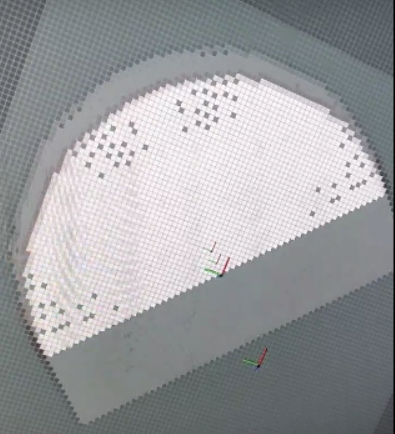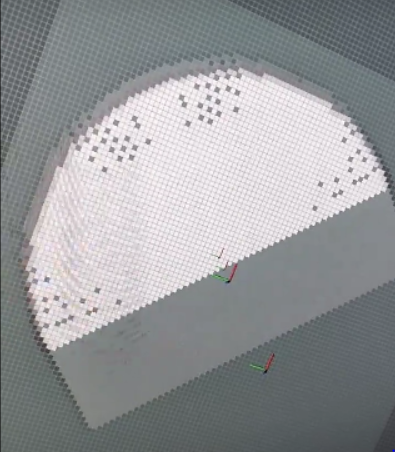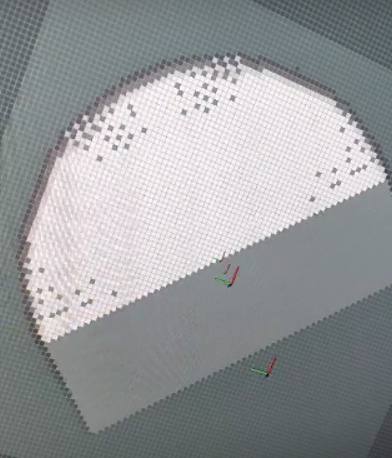即重合了。
五、前端融合算法
主要用于IMU和轮子前端融合，也可以不在前端，直接紧耦合或者在后端融合
5.1、EKF
属于高斯滤波。
之前写过不作过多论述，主要代码实现就是J的写法：
    JF << 1.0,1.0,-dt*v*sin(yaw),dt*cos(yaw),
0.0,1.0,dt*v*cos(yaw) ,dt*sin(yaw),
0.0,0.0,1.0           ,0.0        ,
0.0,0.0,0.0           ,1.0        ;

5.2、比例融合
这个没啥难的，就是yaw按轮子和imu给的那个按比列分配即可。
六、后端融合算法
主要有4种，本还想加入SEIF，IF主要的优势是不知道权重Omega给0即可，而KF协方差要给无限大，不过现在主要图优化时代，SEIF实际用的少。其实这个我之前就写过了，见我之前写的，写过我就不说了。
6.1、EKF
就上面讲过，关键是imu，轮子，匹配的误差模型，imu误差模型 ，轮子误差模型就是av×v + b×W×W，这个在概率机器人中说的很明白了。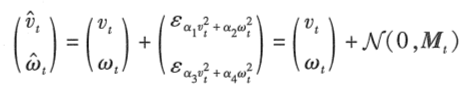激光雷达误差模型一是概率机器人波束模型那样算，二是直接按分数，归一化后×length即可。
6.2、UKF
这个我之前也写了，就取2n+1个点变换过去，再重新计算均值和方差即可，可见我之前写的UKF。现实情况如果误差模型建的好， 其误差残项十分符合高斯分布，那么UKF好于EKF，否则则不一定
6.3、PF
这个我也写过，particle filter，粒子滤波，gmapping用的是改进版粒子滤波，其在预测粒子生成后还进行了前后左右，左转右转6次的CSM分数探测，取了分数最大的那个，这样预测粒子分布就好了很多，不过严重依赖里程计，说白了就是6次探测跟不不够，有时候误差高达10个栅格长度，那就没戏了要么加大粒子数，不过因为gmapping一个粒子维持一个地图，其内存和计算量都会变得十分大，这也导致了gmapping在数据集处理旋转很好，但是实际运用过程中还是受不了不停旋转，比如intel数据集下表现很好，但是你拿到实际场景就不行，效果甚至不如ICP，（说ICP过时了的可以看ICP+RANSAC+图优化论文，scan to map是担心scan to scan的累计误差，但加入图优化就得到很好的解决）最后gmapping 用Neff统计粒子分散度，如果过于分散就重采样，但是重采样是个败笔，数据集中可以，比如MIT那个数据集，但是实际过程中，重采样往往整个位姿就坏了。
然后在实现过程中，按权重采样可以按如下实现,使用 random库：
//之前是 W += count W，所以每个粒子占有一个区间，随机出来粒子看在哪个区间就取哪个粒子

std::default_random_engine e;
e.seed(time(NULL));
std::uniform_int_distribution<unsigned> nw(0, W);
for(U4 i = 0;i<param.x_set_size;i++){
I4 wp = nw(e);
R8 low_b = 0;
for(U4 j = 0;j<x_pred_set.size();j++){
if(wp<x_pred_set[j].Wc && wp >= low_b){
x_set.push_back(x_pred_set[j]);
break;
}
low_b += x_pred_set[j].Wc;
}
}

6.4、Graph Optimizer
图优化算法是cartographer的主要核心，见图优化，主要就是把误差项填入边，然后按SPA求解稀疏矩阵即可，求解的时候按如下：
Xx = Hx.colPivHouseholderQr().solve(bx);

也可用LU分解，或者Cholesky，LLT都可以，普通的边就是当前位姿与最近距离小于dis且索引小于n的点，而回环边就是那些不是最近索引点的距离与当前匹配位姿小于dis的点，回环优化后地图要重新生成，并把回环边去掉，如下：
void Fusion::Reconstruct_Map(Mapper &mapper,Mapper_Param &mapper_param,Fusion_Param & fusion_param){
mapper.multi_map.clear();
mapper.Init_Map(node_set,scan_set,mapper_param);
for(I4 i = 1;i<node_set.size();i++){
mapper.Get_Local_Map(node_set[i],scan_set[i],mapper_param);
mapper.Mix_Local_Map_With_SBF(mapper_param);
}
for(I4 i = 0;i<extra_edge;i++){
edge_set.pop_back();
}
}

而优化可见lsslamPDF，激光slam和视觉不同的在于，激光slam不需要用DBow3或者深度学习回环，但不代表不可以，你用这个添加环也是可以的，cartographer中用ceres优化自动求导，当然如果你懂雅可比矩阵求导的话，为了效率建议用Eigen。有些人觉得图优化要优化那么多速度肯定很慢，其实不然，如果是加入landmark的graph确实慢，但现在是Pose graph只有node，所以速度不比gmapping慢，内存也没gmapping消耗高。其保存形式如下：

class Pose_Edge{
public:
Pose_Edge();
~Pose_Edge();

U4 x_i;
U4 x_j;
Pose_2d edge;
Pose_2d omega;
};
std::vector<Pose_Edge> edge_set;
std::vector<Pose_2d> node_set;
std::vector<std::vector<Pose_2d>> scan_set;

故其实消耗内存也很少,最终效果如下：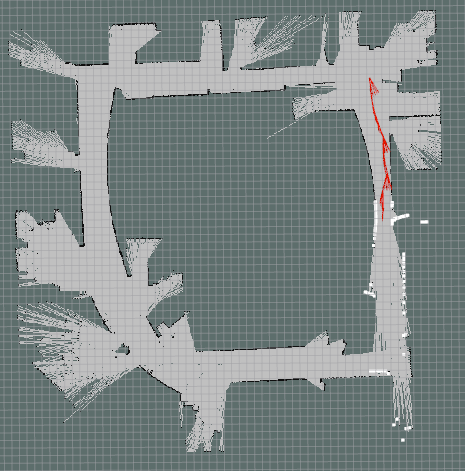展开全文• 激光雷达2D SLAM基本框架1 1.前端：传感器获取数据和扫描匹配 2.后端：优化和闭环检测 3.地图创建 扫描匹配 1.基于点的扫描匹配 ICP算法 寻找一致点对=>剔除冗余点对=>根据对应点计算RT=>点云转换，计算...
• <p>Hello, <p>It's taking forever to produce a refine map for cartographer. I am tuning it for days but the results are... I am using odometry and 2d lidar. Below is the screen shot for cartographer: ...
• cartographer通过main()函数调用void Run()来启动程序，Run()也承接了cartographer的业务逻辑。 void Run() { constexpr double kTfBufferCacheTimeInSeconds = 10.; //tf2_ros::Buffer是tf2 library的主要工具。...
• <p>in offboard mode, to get a perfect drone Pose, how can I integrate the slam_out_pose (of hector_slam, for example) with the /mavros/local_position/pose (which, if I understand well, it'...
• 2D SLAMA. Map Access It should be noted that the sample points/grid cells of the map are situated on a regular grid with distance 1 (in map coordinates) from each other, which simpliﬁes the...